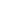Flat 50% off

Ends in

## Answer the question 1.4Coulomb's law for electrostatic force between two point charges and Newton's law for gravitational force between two stationary point masses, both have inverse-square dependence on the distance between the charges/masses. (a) Compare the strength of these forces by determining the ratio of their magnitudes (i) for an electron and a proton and (ii) for two protons. (b) Estimate the accelerations of electron and proton due to the electrical force of their mutual attraction when they are 1 $\overset{\circ}{\mathrm {A}}$ (= $10^{-10}$ m) apart? $(m_p = 1.67 \times 10^{-27}\ kg, m_e = 9.11 \times 10^{-31}\ kg)$

### Asked By Mofiz Ali

Updated Wed, 12 Dec 2018 04:17 pm